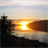# QlikView App Dev

Discussion Board for collaboration related to QlikView App Development.

Announcements
Now Live: Qlik Sense SaaS Simplified Authoring – Analytics Creation for Everyone: READ DETAILS
cancel
Showing results for
Did you mean:Contributor II

## Optimize expression

Hello Users,

Is there any way to optimize the below sum if /set analysis expression

The below expression works well in charts... need to replace with an optimized expression which consumes less RAM .

Is there any way you can fine tune below expression?

`sum({<Date*={">\$(vStart)<\$(vEnd)"}>}Sales* if((1-(fabs(Sales-Estimate))/Sales)<0,0,(1-(fabs(Sales-Estimate))/Sales)))/sum({<Date*={">\$(vStart)<\$(vEnd)"}>} Sales)`

I dont want to do it in scripts... so prefer it in expressions. So can someone help me with this...

Labels (3)

• ### sumif

1 Solution

Accepted SolutionsMVP

May be this

```Sum({<Date*={">\$(vStart)<\$(vEnd)"}>}Sales * RangeMax((1-(fabs(Sales-Estimate))/Sales), 0))
/
Sum({<Date*={">\$(vStart)<\$(vEnd)"}>} Sales)``````Sum({<Date*={">\$(vStart)<\$(vEnd)"}>}Sales * RangeMax((1-(fabs(Sales-Estimate))/Sales), 0))